# Universal sequence map (USM) of arbitrary discrete sequences

## Abstract

### Background

For over a decade the idea of representing biological sequences in a continuous coordinate space has maintained its appeal but not been fully realized. The basic idea is that any sequence of symbols may define trajectories in the continuous space conserving all its statistical properties. Ideally, such a representation would allow scale independent sequence analysis – without the context of fixed memory length. A simple example would consist on being able to infer the homology between two sequences solely by comparing the coordinates of any two homologous units.

### Results

We have successfully identified such an iterative function for bijective mappingψ of discrete sequences into objects of continuous state space that enable scale-independent sequence analysis. The technique, named Universal Sequence Mapping (USM), is applicable to sequences with an arbitrary length and arbitrary number of unique units and generates a representation where map distance estimates sequence similarity. The novel USM procedure is based on earlier work by these and other authors on the properties of Chaos Game Representation (CGR). The latter enables the representation of 4 unit type sequences (like DNA) as an order free Markov Chain transition table. The properties of USM are illustrated with test data and can be verified for other data by using the accompanying web-based tool:http://bioinformatics.musc.edu/~jonas/usm/.

### Conclusions

USM is shown to enable a statistical mechanics approach to sequence analysis. The scale independent representation frees sequence analysis from the need to assume a memory length in the investigation of syntactic rules.

## Background

For over a decade the idea of representing biological sequences in a continuous coordinate space has maintained its appeal but not been fully realized . The basic idea is that sequences of symbols, such as nucleotides in genomes, aminoacids in proteomes, repeated sequences in MLST [Multi Locus Sequence Typing, 4], words in languages or letters in words, would define trajectories in this continuous space conserving the statistical properties of the original sequences [3, 59]. Accordingly, the coordinate position of each unit would uniquely encode for both its identity and its context, i.e. the identity of its neighbors . Ideally, the position should be scale-independent, such that the extraction of the encompassing sequence can be performed with any resolution, leading to an oligomer of arbitrary length. The pioneer work by Jeffrey published in 1990  achieved this for genomic sequences by using the Chaos Game Representation technique (CGR), defining a unit-square where each corner corresponds to one of the 4 possible nucleotides. Subsequent work further explored the properties of CGR of biological sequences, but two main obstacles prevented the realization of its early promise – lack of scalability with regard to the number of possible unique units and inability to represent succession schemes. Meanwhile, Markov Chain theory already offered a solid foundation for the identification of discrete spaces to represent sequences as cross-tabulated conditional probabilities – Markov transition tables. This Bayesian technique is widely explored in bioinformatic applications seeking to measure homology and align sequences . In a recent report  we have shown that, for genomic sequences, Markov tables are in fact a special case of CGR, contrary to what had been suggested previously . This raised the prospect of an advantageous use of iterative maps as state spaces not only for representation of sequences but also to identify scale independent stochastic models of the succession scheme. That work  is hereby extended and further generalized to be applicable to sequences with arbitrary numbers of unique component units, without sacrificing the inverse correlation between distance in the map and sequence similarity independent of position. Accordingly, the technique is named Universal Sequence Map (USM).

## Results

The Results are divided in two sections. The first section presents the foundations for identifying an iterative function with the desired properties. The second section describes algorithm implementation illustrated with a sample data set. Both sections are best understood by using the accompanying web-based tool (see Abstract for address) where the different steps of the procedure can be verified and reproduced with the test data or the reader's own data.

### Conceptual foundations

The USM generalization proposed here is achieved by observing two stipulations: A-alternative units in the iterative map are positioned in distinct corners of unit block structures', and B – sequence processing is bi-directional.

Basis for USM generalization:

1. A.

Each unique unit is referenced in the map for positions that are at equal n-distances from each other, and possibly, but not necessarily, defining a complete block structure. n-distances are defined as the maximum distance along any dimension, e.g. n-distance between [a1, a2, ...,a n ] and [b1, b2, ...,b n ] is max(|b1 - a1|, |b2- a2|, ..., |b n - a n |), see also Equation 3. It will be shown that this stipulation leads to the definition of spaces where distance is inversely proportional to sequence similarity, independent of position. In this respect, USM departs from previous attempts to generalize Chaos Game Representation that conserve the bi-dimensionality of the original CGR representation [8, 1517].

2. B.

The iterative positioning is performed in both directions. Therefore, there will be two sets of coordinates, the result of forward and backward iterative operations. It will be shown that, by adding backward and forward map distances between two positions, the number of identical units in the encompassing sequences can be extracted directly from the USM coordinates. As a consequence, two arbitrary positions can be compared, and the number of contiguous similar units is extracted by an algebraic operation that relies solely on the USM coordinates of those very two positions.

### Implementation of USM algorithm

The algorithm will be first illustrated for the first and last stanzas of Wendy Cope's poem "The Uncertainty of the Poet" (14), respectively, "I am a poet. I am very fond of bananas." and "I am of very fond bananas. Am I a poet?". The procedure includes four steps:

1. 1.

Identification of unique sequence units – e.g. these two stanzas have 19 unique characters, (table 1), i.e. uu = 19.

2. 2.

Replacement of each unique unit (in this case units are alphabetic characters) by a unique binary number – e.g. in table 1 each of the 19 unique units is replaced by its rank order minus one, represented as a binary number. Other arrangements are possible leading to the same final result as discussed below. The minimum number of dimensions necessary to accommodate uu unique units, n, is the upper integer of the length of its binary representation: n = ceil(log2(uu)). For W. Cope's stanzas, n = ceil(log2(19)) = 5. The binary reference coordinates for the unique units are defined by the numerals of the binary code – for example, a will be assigned to the position U'a' = [0,0,1,0,1]. Each symbol is represented as a corner in a n-dimensional cube (Table 1). The purpose of these first two steps is to guarantee that the reference positions for each unique sequence unit component are equidistant (stipulation A) in the n-metric defined above. Any other procedure resulting in equidistant unique positions will lead to the same final results independently of the actual binary numbers used or the number of dimensions used to contain them.

1. 3.

The CGR procedure  (Eq. 1) is applied independently to each coordinate, j = 1,2, ...,n, for each unit, i, in the sequence of length k, u j (i) with i = 1,2, ...,k, and starting with a random map position taken from a uniform distribution in [0,1]n, i.e. Unif([0,1]n). The random seed is not fundamentally different from using the middle position in the map as is conventional in CGR and it has the added feature that it prevents the invalidation of the inverse logarithmic proportionality of n-distance to sequence similarity  for sequences that start or end with the same motif.

For a sequence with k units, the USM positions i = 1,..., k for the j = 1,..., n dimensions are determined as follows: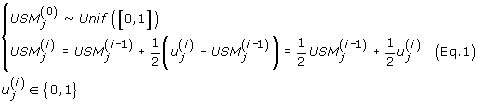1. 4.

The previous step generated k positions in a n-dimension space by processing the sequence forward (Eq. 1). This subsequent step adds an additional set of n dimensions by implementing the same procedure backward (Eq. 2), again starting at random positions for each coordinate. Consequently the first n dimensions of USM will be referred as defining a forward map and the second set of n dimensions will define a backward map. Put together, the bidirectional USM map defines a 2n-unit block structure.

The n additional backward coordinates are determined as follows: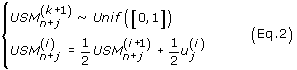The forward USM map for genomic sequences, where uu = 4, and, consequently, n = 2, is the same as the result generated by CGR. However, by freeing the iterative map from the dual-dimensional constraint of conventional CGR, the USM forward map alone achieved the goal of producing a scale independent representation of sequences of arbitrary number of unique units. These properties will be briefly illustrated with W Cope's example. The 16th unit of the first stanza, "I am a poet. I am very fond of bananas.", has USM coordinates USM [1,...,2n] (16) = [0.02 0.01 0.63 0.00 0.53 0.07 0.30 0.52 0.27 0.57]. The first n = 5 coordinates, the position in the forward map, can now be used, by reversing equation 1 [12, 13], not only to extract the identity the unit i = 16 but also the identity of the preceding units:

• using forward coordinates alone [0.0156 0.0138 0.6314 0.0001 0.5338]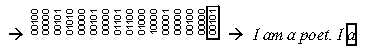The same procedure can be applied to the remaining n = 5 coordinates, the position in the backward map, to extract the identity of the succeeding units, now ordered backwards.

• using backward coordinates alone [0.0703 0.3004 0.5169 0.2742 0.5652]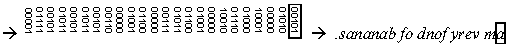The length of the sequence that can be recovered from a position in the CGR or USM space is only as long as the resolution, in bits, of the coordinates themselves. In addition, the relevance of these iterative techniques is not associated with the property of recovering sequences as much as with the ability to recover the succession schemes, e.g. the Markov probability tables. It has been recognized for almost a decade that the density of positions in unidirectional, bi-dimensional, iterated CGR maps (e.g. of genomic sequences, uu = 4 ->n = 2) defines a Markov table [12, 13]. The complete accommodation of Markov chains in unidirectional USM (i.e. either forward or backward, which is an equivalent to a multidimensional solution for CGR) can be quickly established by noting that the identity of a quadrant is set by its middle coordinates. In order to extract the Markov format, for an arbitrary integer order ord, each of the two n-unit hypercubes, the set of n forward or backward coordinates, would be divided in q = 2n.(ord+1) equal quadrants and the quadrant frequencies rearranged . The use of quadrant to designate what is in fact a sub-unit hypercube is a consonance with the preceding work on bidimensional CGR maps , where it was shown that since any number of subdivisions can be considered in a continuous domain, the density distribution becomes an order-free Markov Table that accommodates both integer and fractal memory lengths. The extraction of Markov chain transition tables from USM representations, both forward and backward, is included in the accompanying web-based application (see Abstract).

Above, the USM procedure was shown to allow for the representation of sequences as multidimensional objects without loss of identity or context. These objects can now be analyzed to characterize the sequences for quantities such as similarity between segments or entropy [18, 19] within the sequence. In figure 1 the 10-dimensional object defined by the USM positions of the two stanzas was projected in 3-dimensions by principal component analysis. The dimensionality reduction by principal factor extraction has visualization purposes only. As established above, the minimum necessary dimensionality of the USM state space is set by the binary logarithm of the number of unique units. Nevertheless, the sequence variance associated with each component is provided in the figure legend. In figure 1a, the segments " very fond of" in the two stanzas are linked by solid lines to highlight the fact that sequence similarity is reflected by spatial proximity of USM coordinates. The representation is repeated in Figure 1b with solid lining of the segment " bananas". The matching of the two segments of the second stanza (light) to the similar segments of the first stanza (dark) is, again, visually apparent.

The USM algorithm determines that similar sequences, or segments of sequences, will have converging iterated trajectories: the distance will be cut in half for every consecutive similar unit. This property was notice before for CGR of genomic sequences , and will be further explored here for USM generalization. In that preceding work it was shown that the number of similar consecutive units can be approximated by a symmetrical logarithmic transformation of the maximum distance between two positions in either of the dimensions (n-distance), d.

d = -log2 (Max|ΔUSM undirectional |)     (Eq. 3)

Since the USM coordinates include two CGR iterations per dimension, one forward and another backward, two distances can be extracted. The first 1,..., n coordinates define a forward similarity estimate, d f , and the second n+1,..., 2n coordinates can be used to estimate backward similarity, d b . The former measures similarity with regard to the units preceding the one being compared and the latter does the same for those the succeeding that same units. Therefore, the forward and backward distances between the positions i and j of two sequences, a and b, with a length of k a and k b , respectively, would be calculated as described by equation 4, defining two rectangular matrices, d f and d b , of size k a × k b (Fig. 2a,2b).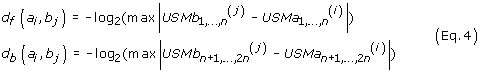However, the values of d necessarily overestimate the number of similar contiguous units preceding (d f , illustration for stanza comparison in Fig. 2a) or succeeding (d b , illustration for stanza comparison in Fig. 2b) the positions being compared. The value of d would be the exact number of contiguous similar units, h, if the starting positions for the similar segments where at a n-distance of 1, e.g. if they were in different corners of the unit hyper-dimensional USM cube. Since the initial distance is always somewhat smaller, the homology, h, measured as the number of consecutive similar units, will be smaller than d (Eq. 5).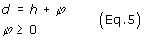The contribution of φ to the similarity distance, d, can be estimated from the distribution of positions in the USM map of a random sequence. A uniformly random sequence [3, 19, 20] will occupy the USM space uniformly, and, for that matter, so will the random seed of forward and backward iterative mapping, respectively equations 1 and 2. Therefore, a uniform distribution is an appropriated starting point to estimate the effect of (p, the over-determination of h by d (Eq.5). Accordingly, for a given x [0,1], the probability, P o , that any two coordinates, x1 and x2, are located within a radius r (0,1) is given by Equation 6.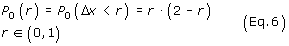Since P o (r) is the probability of two points chosen randomly from a uniform distribution Unif([0,1]) being at a distance less than r from each other, for any set of n coordinates in the USM, the likelihood of finding another position within a block distance of r would be described by raising equation 6 to the n exponent. Finally, recalling from equation 3 that sequence similarity can be obtained by a logarithmic transformation of r, the probability that the unidirectional coordinates of two random sequences are at a similar length d > φ is described by equation 7. The simplicity of the expansion for higher dimensions highlights the order-statistics properties  of the n-metric introduced above (Eq. 3). It is noteworthy that the model for the likelihood of over-determination is the null-model, e.g. the comparison of actual sequences is evaluated against the hypothesis that the similarity observed happened by chance alone.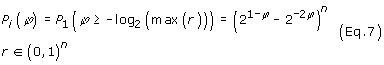Finally, it is also relevant to recall that the null model for d (Eq.7 for unidirectional comparisons, bi-directional null models are derived below) allows the generalization for non-integer dimensions. For example, the 19 unique unites found in the two stanzas (Table 1), define forward and backward USM maps in 5 dimensions each. However the 5th dimension is not fully utilized, as that would require 25 = 32 unique units. Therefore, if there is no requirement for an integer result, the effective value of n for the two stanzas can be refined as being n = log2(19) = 4.25.

An estimation of bi-directional similarity will now be introduced that adds the forward and backward distance measures d f and d b . The motivation for this new estimate is the the determination of the similar length of the entire similar segment between two sequences solely by comparing any two homologous units. Accordingly, since d f is an estimate of preceding similarity and d b provides the succeeding similarity equivalent the sum of the two similar distances, D, (Eq. 8) will estimate of the bi-directional similarity, e.g. the length of the similar segment, H.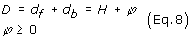As illustrated later in the implementation, for pairwise comparisons of homologous units of similar segments, all values of D and, consequently, of φ, are exactly the same. This result could possibly have been anticipated from the preceding work  by noting that the value of d between two adjacent homologous units differs exactly by one unit. However, this result was in fact a surprise and one with far reaching fundamental and practical implications.

Similarly to unidirectional similarity estimation, d, the bi-directional estimate, D, being the sum of two overestimates, is also overestimated by a quantity to be defined, φ (Eq. 8). The derivation of an expression for the bi-directional overestimation will require the decomposition of P1 (Eq. 7) for two cases, comparison between unidirectional coordinates of similar quadrants, P1a, and of opposite quadrants, P1b, as described in equation 9. Recalling from equation 2, positions in the same quadrant correspond to sequence units with the same identity, and positions in opposite quadrants correspond to comparison between coordinates of units with a different identity.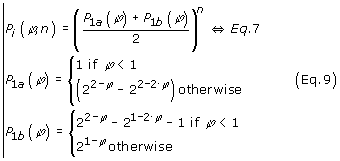The need for the distinction between same and opposite quadrant comparison, which is to say between similar and between dissimilar sequence units, is caused by the fact that same quadrant comparisons are more likely to lead to higher values of d. As illustrated above for the 16th unit of the first stanza, the forward and backward coordinates must fall in the same quadrant. Consequently, the similar pattern of same and opposite quadrant comparisons for each dimension will be reflected as a bias in the bi-directional overestimation. The determination of probability, P2, of over-determination between sums of independent unidirectional similarity estimates is derived in equation 10.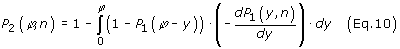The probability of bi-directional over-determination, can now be established by using the same and opposite unidirectional comparison expressions presented in Equation 9. The resulting expression for similarity over-determination by the distance between bidirectional USM coordinates, P3, is presented in equation 11.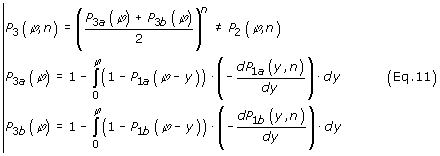In figure 3, the probability distribution for both unidirectional (P1, in gray) and bidirectional (P3, in black) comparisons is represented for different dimensions, n. It is clearly apparent that the over-determination becomes much less significant as dimensionality increases. From a practical point of view, the over-determination is of little consequence because the computational load of comparing sequences corresponds mostly to the identification of candidate pairing combinations. The fact that the n-metric unidirectional distances, d f and d b , defined in Equation 4, and bidirectional D, defined in Eq. 8, are over-determined implies that the identification of similar segments between two sequences will include false positives but will not generate false negatives. The false positive identifications can be readily recognized by comparing the sequences extracted from the coordinates, as demonstrated above for the 16th unit of the first stanza. Nevertheless, since over-determination will necessarily occur, its probability distribution was identified (Eq. 11, Fig. 3). This can also be achieved for individual values by solving Eq. 11 for the value of φ observed. For example, for the conditions of the two stanzas, the value of (φp 1 = 0.5, n = 4.25 is 0.71 sequence units, which is the expected median unidirectional over-determination, P1, of d f and d b (Eqs. 5, 7). The corresponding probability of bi-directional overdetermination, P3, should be somewhat above twice that value. Using equation 11, the value obtained is 1.67 similar units. Finally, it is worthy to stress that the expressions for calculation of likelihood of arbitrary levels of over-determination (Eq. 5–11) can be inverted to anticipate the level of over-determination for arbitrary probability levels. This use of the null random model is also included in the accompanying online tool (see Abstract for URL).

## Discussion

H is the number of contiguous units that are similar between the two sequences aligned at the positions being compared (Eq. 8). This value is estimated by D, which is the sum of the overestimated number of preceding, d f , and succeeding, d b , homologous units (Eq. 4, 5 and 8). The determination of these similarity estimates, d f and d b , was illustrated for the two stanzas in figures 2.a,2b. The same values compensated for over-determination at P3 = 0.5 are represented in Fig. 2c. The striking property of bi-directional similarity (H, Eq.8) is that the D values obtained for any two homologous pair from similar segments are exactly the same. That value is an estimator of the length of the entire similar segment, H (Eq. 11). This is further illustrated in figure 5 for comparison of genomic sequences, where it is also observed that the values of the distances between similar segments are constant and estimate the similar length. This was a somewhat unexpected property of enormous practical value since the length of the similar segment can be determined by a single pair-wise comparison between any of analogous positions. Consequently, when comparing two sequences of length k a and k b to identify all similar segments of length w or above, k a k b /w pair-wise comparisons will suffice. In addition, each pair-wise comparison is now achievable with a single algebraic operation (Eq.8) rather than requiring the conventional dynamic programming approach . The computational effort of positioning database sequences in the USM state space occurs at the level of database indexing. Consequently, search algoritms based on the USM state space representation will necessarily lead to speedier implementations. In order to facilitate the comparison with dynamic programming, the software library of functions, in MATLAB format, Mathworks Inc., for the determination of USM coordinates is also provided http://bioinformatics.musc.edu/~jonas/usm/.

Additional measures of similarity can be derived for specific practical purposes using bi-directional and unidirectional d values. For example, the use of docking algorithms to align sequences would benefit from a measure with a maximum value in the center of the similar segments. This could be provided by defining a compounded similarity measure, Hc, as suggested in equation 12. The behavior of Hc, which would be obtained by the overestimated value of dc, is illustrated for the two test stanzas in Figure 2.d.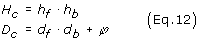The detection of similar segments in arbitrary sequences using D becomes very effective as the length of the similar segment increases. This was clear in the distribution of over-determination in Fig. 3 but it is even more so when the distances between sequences with homologous segments are represented. In figure 4 the distances between the two stanzas are represented alongside the distances to be expected if no homology existed, apart from the coincidental (random null model, using Eq. 11). It can be observed for the comparison of the two stanzas (Fig. 4, gray lines) that H values above 4 units occur with higher frequency than allowed by the random distribution model, reflecting the presence of real homologous segments (similar words).

### USM of biological sequences

The representation of biological information as discrete sequences is dominated by the fact that genomes are sequences of discrete units and so are the products of its transcription and translation. However, not all biological sequences are composed of units that are functionally equally distinct from each other, as is the case of proteomic data and Multi-locus sequence typing [MLST, 4]. To avoid the issue of unit inequality and highlight the general applicability of the USM procedure, stanzas of a poem were used to illustrate the implementation instead. Nevertheless the original motivation of analyzing biological sequences is now recalled.

In the preceding report the authors have illustrated the properties of unidirectional n-metric estimation of similarity for the threonine operon of E. coli. The same two two regions of thrA and thrB sequences of E. coli K-12 MG1655 are compared in Figure 5 to highlight the advancement achieved by USM. It should be recalled that the particular dimensionality of DNA sequences, n = 2, allows a very convenient unidirectional bi-dimensional representation, which is in fact the Chaos Game Representation procedure (CGR) . Consequently, CGR is a particular case of USM, obtained when n = 2 and only the forward coordinates are determined. This can also be verified by comparing Figure 5a with a similar representation reported before , obtained with the same data using CGR [Fig. 10 of that report]. The advantageous properties of full (bi-directional) USM become apparent when Fig. 5a is compared with Fig. 5b. It is clearly apparent for bi-directional USM (Fig. 5b) that all pair-wise comparisons of units of identical segments now have the same D values. This coverts any individual homologous pair-wise comparison into an estimation of the length of the entire similar segment. The conservation of statistical properties by the distances obtained, D, can also be confirmed by comparing observed values with the corresponding null models (Fig. 4). For the analysis of this figure it is noteworthy to recall that the statistical properties of prokaryote DNA are often undistinguishable from uniform randomness [12, 18, 19]. The genomic sequence of the first gene of the threonine operon of E. coli, thrA, is compared with that of the second, thrB. The distribution of the resulting D values is represented in figure 4 (solid black line), alongside with the null model for that dimensionality (Eq. 11, with n = log2(4) = 2, gray dotted line). The genomic sequences of thrA and thrB were translated into proteomic sequences using SwissProt's on line translator, applied to the 5'3' first frame http://www.expasy.ch/tools/dna.html. Similarly, the distribution of D values for the comparison of the proteomic thrA and thrB sequences is also represented in Figure 4, alongside with the null model, Eq. 11, for its dimensionality (n = log2(uu = 20 possible aminoacids) = 4.32), which is graphically nearly undistiguishable from that of the comparison between the stanzas, with n = log2(uu = 19 possible letters) = 4.25 (dotted gray line for the rounded value, n = 4.3). Both the genomic and the proteomic distribution of D values is observed to be contained by the null model, unlike the comparison between the stanzas discussed above, where the existence of structure is clearly reflected by its distribution. The genomic and proteomic of thrA and thraB, used to illustrate this discussion, are provided with the web-based implementation of USM (see Methods for URL).

## Conclusions

The mounting quantity and complexity of biological sequence data being produced  commands the investigation of new approaches to sequence analysis. In particular, the need for scale independent methodologies becomes even more necessary as the limitations of conventional Markov chains are increasingly noted . These limitations are bound to become overwhelming when signals such as succession schemes of the expression of over 30,000 human genes  become available. This particular signal would be conveniently packaged within a 30 dimension USM unit block (n = ceil(log2(3 103) = 15).

In addition, the advances in statistical mechanics for the study of complex systems, particularly in non-linear dynamics, have not been fully utilizable for the analysis of sequences due to the missing formal link between discrete sequences and trajectories in continuous spaces. The properties of USM reported above suggest that this may indeed be such a bridge. For example, the embedding of dimensions, a technique at the foundations of many time-series analysis techniques offers a good example of the completeness of USM representation of sequences. By embedding the forward and backward coordinates separately, at the relevant memory length, the resulting embedded USM is exactly what would be obtained by applying USM technique to the embedded dimeric sequence itself.

## Methods

### Computation

The algorithms described in this manuscript were coded using MATLAB™ 6.0 language (Release 12), licensed by The MathWorks Inc http://www.mathworks.com. An internet interface was also developed to make them freely accessible through user-friendly web-pages http://bioinformatics.musc.edu/~jonas/usm/.

### Source code

In order to facilitate the development of sequence analysis applications based on the USM state space, the software library of functions written to calculate the USM coordinates is provided with the web-based implementation (see address above). The code is provided in MATLAB format, which is general enough as to be easily ported into other environments. These functions process sequences provided as text files in FASTA format. In addition to the functions, the test datasets and a brief readme.txt documentation file are also included.

### Test data

The USM mapping proposed is applicable to any discrete sequence, even if the primary goal is the analysis of biological sequences. For ease of illustration and to emphasize USM's general validity, the test dataset used to describe implementation of the algorithm consists of two stanzas of a Poem by Wendy Cope, "The Uncertainty of the Poet" . In the Discussion section, USM was also applied to the DNA sequence of the threonine operon of Escherichia coli K-12 MG1655, obtained from the University of Winsconsin E. coli Genome Project http://www.genetics.wisc.edu, and to its 5'3' first frame proteomic translation obtained by using SwissProt on line translator http://www.expasy.ch/tools/dna.html. The three test sequence datasets are also included in the web-based USM application.

## References

1. Román-Roldán R, Bernaola-Galván P, Oliver JL: Application of information theory to DNA sequence analysis: a review. Pattern Recognition 1996, 29: 1187–1194. 10.1016/0031-3203(95)00145-X

2. Nady A: Recent investigations into global characteristics of long DNA sequences. Indian Journal of Biochemistry and Biophysics 1994, 31: 149–155.

3. Tiòo P: Spatial representation of symbolic sequences through iterative function systems. IEEE Transactions on Systems, Man, and Cybernetics – Part A: Systems and Humans 1999, 29: 386–393. 10.1109/3468.769757

4. Enright MC, Knox K, Griffiths D, Crook DWM, Spratt BG: Multilocus sequence typing of Streptococcus pneumoniae directly from cerebrospinal fluid. Eur. J. Clin. Microbiol. Infect. Dis. 2001, 19: 627–630. 10.1007/s100960000321

5. Jeffrey HJ: Chaos game representation of gene structure. Nucleic Acid Res. 1990, 18: 2163–2170.

6. Hill AK, SM Singh: The evolution of species-type specificity in the global DNA sequence organization of mitochondrial genomes. Genome 1997, 40: 342–356. 10.1006/geno.1996.4574

7. Forte B, Mendivil F, Vrscay ER: "Chaos games" for iterated function systems with grey level maps. SIAM J. Math. Anal. 1998, 29: 878–890. 10.1137/S0036141096306911

8. Fiser A, Tusnády GE, Simon I: Chaos game representation of protein structures. Mol. Graphics 1994, 12: 302–304. 10.1016/0263-7855(94)80109-6

9. Deschavanne PJ, Giron A, Vilain J, Fagot G, Fertil B: Genomic sigature: characterization and classification of species assessed by chaos game representation of sequences. Mol. Biol. Evol. 1999, 16: 1391–1399.

10. Roy A, Raychaudhury C, Nandy A: Novel techniques of graphical representation and analysis of DNA sequences – a review. J. Biosci. 1998, 23: 55–71.

11. Durbin R, Eddy S, Krogh A, Mitchison G: Biological Sequence Analysis – Probabilistic Models of Proteins and Nucleic Acids Cambridge University Press, Cambridge 1998.

12. Almeida JS, Carriço JA, Maretzek A, Noble PA, Fletcher M: Analysis of genomic sequences by chaos game representation. J. Bioinformatics 2001, 17: 429–437. 10.1093/bioinformatics/17.5.429

13. Goldman N.: Nucleotide, dinucleotide and trinucleotide frequencies explain patterns observed in chaos game representations of DNA sequences. Nucleic Acids Res. 1993, 21: 2487–2491.

14. Cope W: Serious Concerns, Faber & Faber Inc 1993.

15. Basu S, Pan A, Dutta C, Das J: Chaos game representation of proteins. J. Mol. Graph. Model. 1997, 15: 279. 10.1016/S1093-3263(97)00106-X

16. Pleiβner KP, Wernisch L, Oswald H, Fleck E: Representation of amino acid sequences as two-dimensional point patterns. Electrophoresis 1997, 18: 2709–2713.

17. Solovyev VV, Korolev SV, Lim HA: A new approach for the classification of functional regions of DNA sequences based on fractal representation. Int. J. Genom. Res. 1993, 1: 109–128.

18. Román-Roldán R, Bernaola-Galván P, Oliver JL: Entropic feature for sequence pattern through iterated function systems. Pattern Recognition Letters 1994, 15: 567–573. 10.1016/0167-8655(94)90017-5

19. Oliver JL, Bernaola-Galván P, Guerrero-Garcia J, RomáRolán R: Entropic profiles of DNA sequences through chaos-game-derived images . J. Theor. Biol. 1993, 160: 457–470. 10.1006/jtbi.1993.1030

20. Mata-Toledo RA, Willis M: Visualization of random sequences using the chaos game algorithm. J. Systems Software 1997, 39: 3–6. 10.1016/S0164-1212(96)00158-6

21. Arnold BC, Balakrishnan N, Nagaraja HN: A first course in order statistics. Wiley Series in Probability and Mathematical Statistics: Probability and Mathematical Statistics John Wiley & Sons, Inc., New York 1992.

22. Roos DS: Bioinformatics – trying to swim in a sea of data. Science 2001, 291: 1260–1261. 10.1126/science.291.5507.1260

23. Venter JC, et al.: The sequence of the human genome. Science 2001, 291: 1304–1351. 10.1126/science.1058040

## Acknowledgments

The authors thank Dr Santosh Mishra, Eli Lilly Co., for the insightful suggestions about the applicability of USM, and John H. Schwacke, at the Department of Biometry and Epidemiology of the Medical University of South Carolina for revising the coherence of mathematical deduction. The authors thankfully acknowledge financial support by grant SFRH/BD/3134/2000 to S. Vinga and project SAPIENS-34794/99 of Fundação para a Ciência e Tecnologia of the Portuguese Ministry of Science and Technology.

## Author information

Authors

### Corresponding author

Correspondence to Jonas S Almeida.

## Authors’ original submitted files for images

Below are the links to the authors’ original submitted files for images.

## Rights and permissions

Reprints and Permissions

Almeida, J.S., Vinga, S. Universal sequence map (USM) of arbitrary discrete sequences. BMC Bioinformatics 3, 6 (2002). https://doi.org/10.1186/1471-2105-3-6

• Accepted:

• Published:

• DOI: https://doi.org/10.1186/1471-2105-3-6

### Keywords

• Null Model
• Biological Sequence
• Discrete Sequence
• Memory Length
• Similar Segment